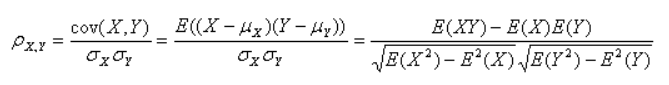# 皮尔逊相关系数python实现

## 一、皮尔逊相关系数## 二、python实现

### 方法1：直接按公式算

``````import numpy as np

x=np.array([1,3,5])
y=np.array([1,3,4])
n=len(x)

sum_xy = np.sum(np.sum(x*y))
sum_x = np.sum(np.sum(x))
sum_y = np.sum(np.sum(y))
sum_x2 = np.sum(np.sum(x*x))
sum_y2 = np.sum(np.sum(y*y))
pc = (n*sum_xy-sum_x*sum_y)/np.sqrt((n*sum_x2-sum_x*sum_x)*(n*sum_y2-sum_y*sum_y))

print(pc)
``````

### 方法2：调用numpy中的corrcoef方法

corrcoef(x, y=None, rowvar=True, bias=np._NoValue, ddof=np._NoValue)

x：array_like，包含多个变量和观测值的1-D或2-D数组，x的每一行代表一个变量，每一列都是对所有这些变量的单一观察。
y：array_like，可选，另外一组变量和观察，y具有与x相同的形状。
rowvar：bool, 可选，如果rowvar为True(默认值)，则每行代表一个变量，并在列中显示。否则，转换关系：每列代表一个变量，而行包含观察。
bias：_NoValue，可选，没有效果，请勿使用
ddof：_NoValue，可选，没有效果，请勿使用

R : ndarray，变量的相关系数矩阵。

``````import numpy as np

x=np.array([1,3,5])
y=np.array([1,3,4])

pc=np.corrcoef(x,y)

print(pc)
``````

### 方法3：调用scipy.stats中的pearsonr方法

pearsonr(x, y)

x：(N,) array_like，Input array。
y：(N,) array_like，Input array。

r : float，皮尔逊相关系数，[-1，1]之间。
p-value : float，Two-tailed p-value（双尾P值）。

``````from scipy.stats import pearsonr
import numpy as np

x=np.array([1,3,5])
y=np.array([1,3,4])

pc = pearsonr(x,y)

print("相关系数：",pc)
print("显著性水平：",pc)
``````

### 方法4：调用pandas.Dataframe中的corr方法

def corr(self,method,min_periods)

method：可选值为{‘pearson’, ‘kendall’, ‘spearman’}
pearson：皮尔逊相关系数
kendall：肯德尔等级相关系数
spearman：斯皮尔曼等级相关系数
min_periods：样本最少的数据量，最少为1。

返回值：各类型之间的相关系数DataFrame表格。

``````import pandas as pd

data=pd.DataFrame({"x":[1,3,5],"y":[1,3,4]})

print(data.corr("pearson"))
``````